Open in App
Not now

# ML | Variational Bayesian Inference for Gaussian Mixture

• Last Updated : 14 Jul, 2019

Prerequisites: Gaussian Mixture

A Gaussian Mixture Model assumes the data to be segregated into clusters in such a way that each data point in a given cluster follows a particular Multi-variate Gaussian distribution and the Multi-Variate Gaussian distributions of each cluster is independent of one another. To cluster data in such a model, the posterior probability of a data-point belonging to a given cluster given the observed data needs to be calculated. An approximate method for this purpose is the Baye’s method. But for large datasets, the calculation of marginal probabilities is very tedious. As there is only a need to find the most probable cluster for a given point, approximation methods can be used as they reduce the mechanical work. One of the best approximate methods is to use the Variational Bayesian Inference method. The method uses the concepts of KL Divergence and Mean-Field Approximation.

The below steps will demonstrate how to implement Variational Bayesian Inference in a Gaussian Mixture Model using Sklearn. The data used is the Credit Card data which can be downloaded from Kaggle.

Step 1: Importing the required libraries

 `import` `numpy as np``import` `pandas as pd``import` `matplotlib.pyplot as plt``from` `sklearn.mixture ``import` `BayesianGaussianMixture``from` `sklearn.preprocessing ``import` `normalize, StandardScaler``from` `sklearn.decomposition ``import` `PCA`

 `# Changing the working location to the location of the data``cd ``"C:\Users\Dev\Desktop\Kaggle\Credit_Card"`` ` `# Loading the Data``X ``=` `pd.read_csv(``'CC_GENERAL.csv'``)`` ` `# Dropping the CUST_ID column from the data``X ``=` `X.drop(``'CUST_ID'``, axis ``=` `1``)`` ` `# Handling the missing values``X.fillna(method ``=``'ffill'``, inplace ``=` `True``)`` ` `X.head()`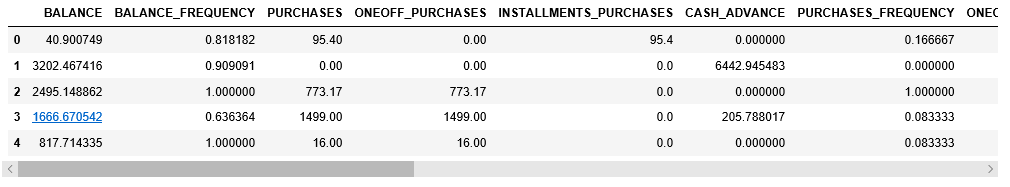Step 3: Pre-processing the data

 `# Scaling the data to bring all the attributes to a comparable level``scaler ``=` `StandardScaler()``X_scaled ``=` `scaler.fit_transform(X)`` ` `# Normalizing the data so that the data``# approximately follows a Gaussian distribution``X_normalized ``=` `normalize(X_scaled)`` ` `# Converting the numpy array into a pandas DataFrame``X_normalized ``=` `pd.DataFrame(X_normalized)`` ` `# Renaming the columns``X_normalized.columns ``=` `X.columns`` ` `X_normalized.head()`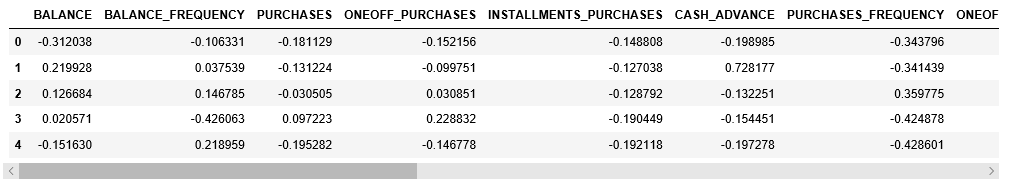Step 4: Reducing the dimensionality of the data to make it visualizable

 `# Reducing the dimensions of the data``pca ``=` `PCA(n_components ``=` `2``)``X_principal ``=` `pca.fit_transform(X_normalized)`` ` `# Converting the reduced data into a pandas dataframe``X_principal ``=` `pd.DataFrame(X_principal)`` ` `# Renaming the columns``X_principal.columns ``=` `[``'P1'``, ``'P2'``]`` ` `X_principal.head()`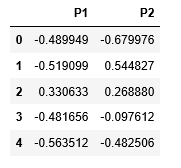The primary two parameters of the Bayesian Gaussian Mixture Class aren_components and covariance_type.

1. n_components: It determines the maximum number of clusters in the given data.
2. covariance_type: It describes the type of covariance parameters to be used.

In the below-given steps, the parameter n_components will be fixed at 5 while the parameter covariance_type will be varied for all possible values to visualize the impact of this parameter on the clustering.

Step 5: Building clustering models for different values of covariance_type and visualizing the results

a) covariance_type = ‘full’

 `# Building and training the model``vbgm_model_full ``=` `BayesianGaussianMixture(n_components ``=` `5``, covariance_type ``=``'full'``)``vbgm_model_full.fit(X_normalized)`` ` `# Storing the labels``labels_full ``=` `vbgm_model_full.predict(X)``print``(``set``(labels_full))`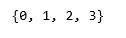`colours ``=` `{}``colours[``0``] ``=` `'r'``colours[``1``] ``=` `'g'``colours[``2``] ``=` `'b'``colours[``3``] ``=` `'k'`` ` `# Building the colour vector for each data point``cvec ``=` `[colours[label] ``for` `label ``in` `labels_full]`` ` `# Defining the scatter plot for each colour``r ``=` `plt.scatter(X_principal[``'P1'``], X_principal[``'P2'``], color ``=``'r'``);``g ``=` `plt.scatter(X_principal[``'P1'``], X_principal[``'P2'``], color ``=``'g'``);``b ``=` `plt.scatter(X_principal[``'P1'``], X_principal[``'P2'``], color ``=``'b'``);``k ``=` `plt.scatter(X_principal[``'P1'``], X_principal[``'P2'``], color ``=``'k'``);`` ` `# Plotting the clustered data``plt.figure(figsize ``=``(``9``, ``9``))``plt.scatter(X_principal[``'P1'``], X_principal[``'P2'``], c ``=` `cvec)``plt.legend((r, g, b, k), (``'Label 0'``, ``'Label 1'``, ``'Label 2'``, ``'Label 3'``))``plt.show()`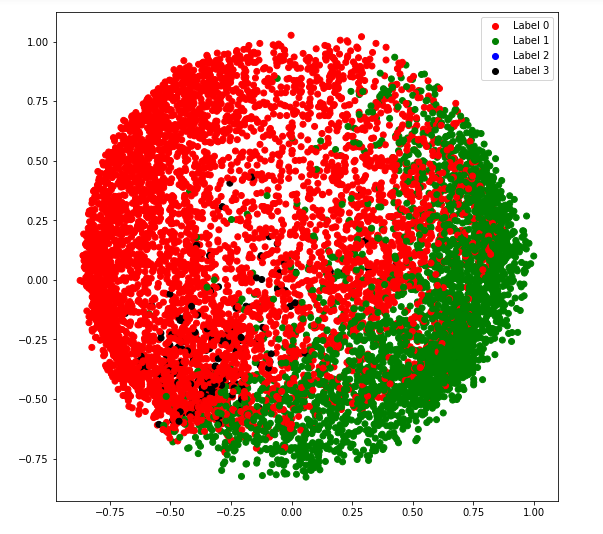b) covariance_type = ‘tied’

 `# Building and training the model``vbgm_model_tied ``=` `BayesianGaussianMixture(n_components ``=` `5``, covariance_type ``=``'tied'``)``vbgm_model_tied.fit(X_normalized)`` ` `# Storing the labels``labels_tied ``=` `vbgm_model_tied.predict(X)``print``(``set``(labels_tied))`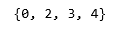`colours ``=` `{}``colours[``0``] ``=` `'r'``colours[``2``] ``=` `'g'``colours[``3``] ``=` `'b'``colours[``4``] ``=` `'k'`` ` `# Building the colour vector for each data point``cvec ``=` `[colours[label] ``for` `label ``in` `labels_tied]`` ` `# Defining the scatter plot for each colour``r ``=` `plt.scatter(X_principal[``'P1'``], X_principal[``'P2'``], color ``=``'r'``);``g ``=` `plt.scatter(X_principal[``'P1'``], X_principal[``'P2'``], color ``=``'g'``);``b ``=` `plt.scatter(X_principal[``'P1'``], X_principal[``'P2'``], color ``=``'b'``);``k ``=` `plt.scatter(X_principal[``'P1'``], X_principal[``'P2'``], color ``=``'k'``);`` ` `# Plotting the clustered data``plt.figure(figsize ``=``(``9``, ``9``))``plt.scatter(X_principal[``'P1'``], X_principal[``'P2'``], c ``=` `cvec)``plt.legend((r, g, b, k), (``'Label 0'``, ``'Label 2'``, ``'Label 3'``, ``'Label 4'``))``plt.show()`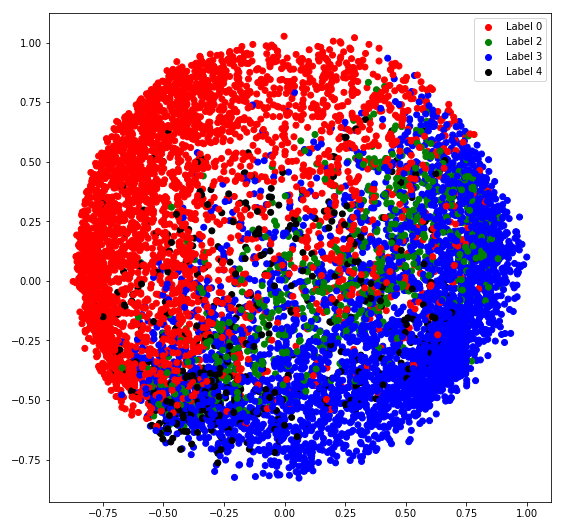c) covariance_type = ‘diag’

 `# Building and training the model``vbgm_model_diag ``=` `BayesianGaussianMixture(n_components ``=` `5``, covariance_type ``=``'diag'``)``vbgm_model_diag.fit(X_normalized)`` ` `# Storing the labels``labels_diag ``=` `vbgm_model_diag.predict(X)``print``(``set``(labels_diag))`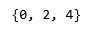`colours ``=` `{}``colours[``0``] ``=` `'r'``colours[``2``] ``=` `'g'``colours[``4``] ``=` `'k'`` ` `# Building the colour vector for each data point``cvec ``=` `[colours[label] ``for` `label ``in` `labels_diag]`` ` `# Defining the scatter plot for each colour``r ``=` `plt.scatter(X_principal[``'P1'``], X_principal[``'P2'``], color ``=``'r'``);``g ``=` `plt.scatter(X_principal[``'P1'``], X_principal[``'P2'``], color ``=``'g'``);``k ``=` `plt.scatter(X_principal[``'P1'``], X_principal[``'P2'``], color ``=``'k'``);`` ` `# Plotting the clustered data``plt.figure(figsize ``=``(``9``, ``9``))``plt.scatter(X_principal[``'P1'``], X_principal[``'P2'``], c ``=` `cvec)``plt.legend((r, g, k), (``'Label 0'``, ``'Label 2'``, ``'Label 4'``))``plt.show()`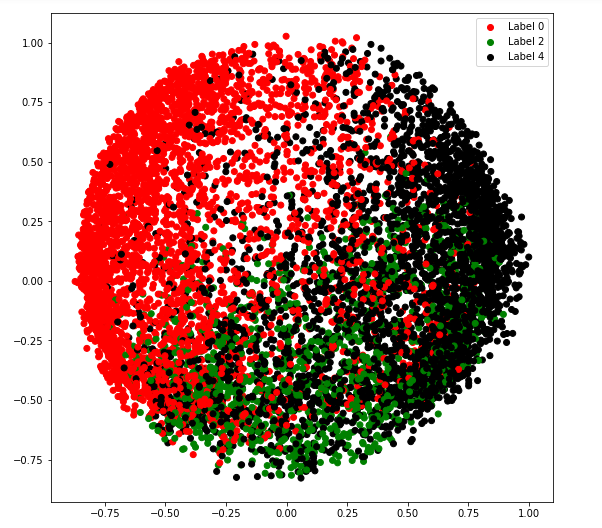d) covariance_type = ‘spherical’

 `# Building and training the model``vbgm_model_spherical ``=` `BayesianGaussianMixture(n_components ``=` `5``,``                                              ``covariance_type ``=``'spherical'``)``vbgm_model_spherical.fit(X_normalized)`` ` `# Storing the labels``labels_spherical ``=` `vbgm_model_spherical.predict(X)``print``(``set``(labels_spherical))`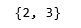`colours ``=` `{}``colours[``2``] ``=` `'r'``colours[``3``] ``=` `'b'`` ` `# Building the colour vector for each data point``cvec ``=` `[colours[label] ``for` `label ``in` `labels_spherical]`` ` `# Defining the scatter plot for each colour``r ``=` `plt.scatter(X_principal[``'P1'``], X_principal[``'P2'``], color ``=``'r'``);``b ``=` `plt.scatter(X_principal[``'P1'``], X_principal[``'P2'``], color ``=``'b'``);`` ` `# Plotting the clustered data``plt.figure(figsize ``=``(``9``, ``9``))``plt.scatter(X_principal[``'P1'``], X_principal[``'P2'``], c ``=` `cvec)``plt.legend((r, b), (``'Label 2'``, ``'Label 3'``))``plt.show()`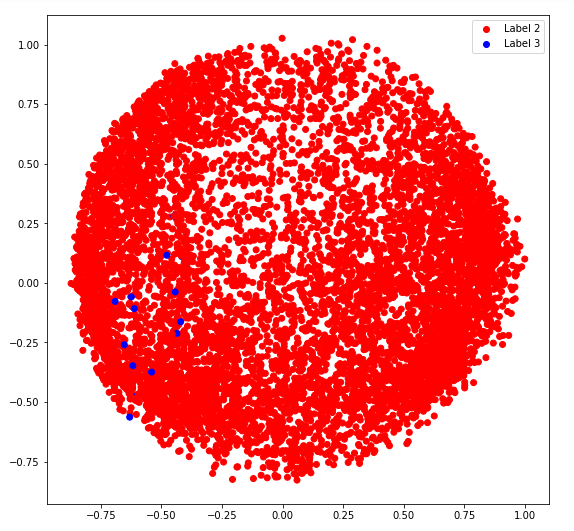My Personal Notes arrow_drop_up